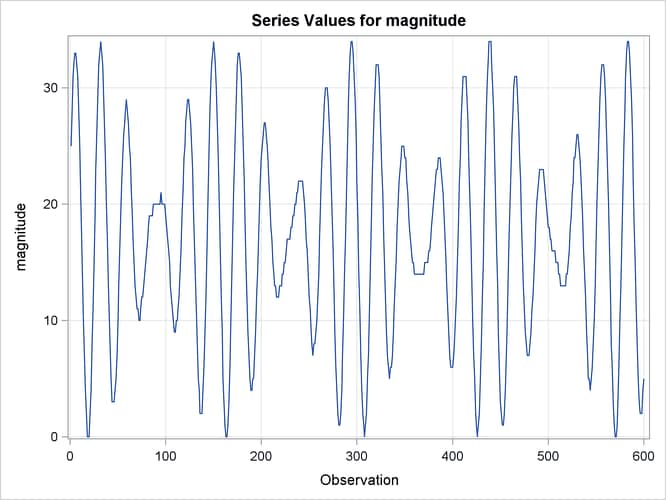Example 35.2 Variable Star Data

The series in this example is studied in detail in Bloomfield (2000). This series consists of brightness measurements (magnitude) of a variable star taken at midnight for 600 consecutive days. The data can be downloaded from a time series archive maintained by the University of York, England (http://www.york.ac.uk/depts/maths/data/ts/welcome.htm (series number 26)). The following DATA step statements read the data in a SAS data set.

```data star;
input magnitude @@;
day = _n_;
datalines;
25  28  31  32  33  33  32  31  28   25   22   18
14  10   7   4   2   0   0   0   2    4    8   11
15  19  23  26  29  32  33  34  33   32   30   27
24  20  17  13  10   7   5   3   3    3    4    5
7  10  13  16  19  22  24  26  27   28   29   28
27  25  24  21  19  17  15  13  12   11   11   10
10  11  12  12  13  14  15  16  17   18   19   19

... more lines ...

```

The following statements use the TIMESERIES procedure to get a timeseries plot of the series (see Output 35.2.1).

```proc timeseries data=star plot=series;
var magnitude;
run;
```

Output 35.2.1: Plot of Star Brightness on Successive DaysThe plot clearly shows the cyclic nature of the series. Bloomfield shows that the series is very well explained by a model that includes two deterministic cycles that have periods 29.0003 and 24.0001 days, a constant term, and a simple error term. He also mentions the difficulty involved in estimating the periods from the data (see Bloomfield 2000, Chapter 3). In his case the cycle periods are estimated by least squares, and the sum of squares surface has multiple local optima and ridges. The following statements show how to use the UCM procedure to fit this two-cycle model to the series. The constant term in the model is specified by holding the variance parameter of the level component to zero.

```proc ucm data=star;
model magnitude;
irregular;
level var=0 noest;
cycle;
cycle;
estimate;
run;
```

The final parameter estimates and the goodness-of-fit statistics are shown in Output 35.2.2 and Output 35.2.3, respectively. The model fit appears to be good.

Output 35.2.2: Two-Cycle Model: Parameter Estimates

The UCM Procedure

Final Estimates of the Free Parameters
Component Parameter Estimate Approx
Std Error
t Value Approx
Pr > |t|
Irregular Error Variance 0.09257 0.0053845 17.19 <.0001
Cycle_1 Damping Factor 1.00000 1.81175E-7 5519514 <.0001
Cycle_1 Period 29.00036 0.0022709 12770.4 <.0001
Cycle_1 Error Variance 0.00000882 5.27213E-6 1.67 0.0944
Cycle_2 Damping Factor 1.00000 2.11939E-7 4718334 <.0001
Cycle_2 Period 24.00011 0.0019128 12547.2 <.0001
Cycle_2 Error Variance 0.00000535 3.56374E-6 1.50 0.1330

Output 35.2.3: Two-Cycle Model: Goodness of Fit

Fit Statistics Based on Residuals
Mean Squared Error 0.12072
Root Mean Squared Error 0.34745
Mean Absolute Percentage Error 2.65141
Maximum Percent Error 36.38991
R-Square 0.99850
Random Walk R-Square 0.97281